## Sunday, January 6, 2019

### Copying a Segment

This applet mimics compass and straightedge constructing on copying a segment. Move the slider to the right to copy segment AB.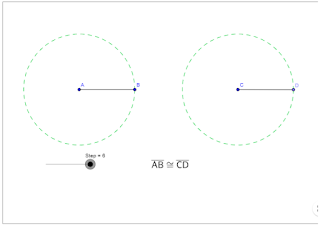## Tuesday, July 3, 2018

### Task 5 - Distance from Point of Similarity

In the figure below, ∆ABC ~ ∆A'B'C' and point P is the point of similarity of the two triangles.

1.) What is the ratio of the lengths of the corresponding sides?

2.) What is the ratio of the distance from point P to the corresponding vertices?

3.) What conjecture can you make from your answer in 1 and 2? Verify your conjectures by drawing a different pair of similar triangles.

Explanation

The ratio of the corresponding sides of two similar triangles is the same as the ratio of the distance of their corresponding vertices to the center of similarity. The ratio of the corresponding sides of two similar triangles is the same as the ratio of the distance of their corresponding vertices. In the figure above, ∆ABC ~ ∆A'B'C' so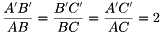and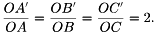## Sunday, July 1, 2018

### Task 4 - Point of Similarity

Shown below are ∆STU and ∆MNO.

Questions

1.) Are the two triangles similar? Why?

2.) What is the ratio of the lengths of their corresponding sides?

3.) Use the Line tool to connect the corresponding vertices of the two triangles. Did your observations in Task 2 and Task 3 hold?

4.) Make a conjecture about the lines connecting the corresponding vertices of similar triangles.

Explanation

If two triangles are similar and their corresponding sides are parallel, then the line connecting their corresponding vertices will intersect at a point. This point is called the center of similarity or point of similarity.

In Task 3, ∆ABC ~ ∆A'B'C' and their corresponding sides are parallel. The three lines connecting the corresponding vertices intersect point P as shown above. In Task 4, ∆STU ~ ∆MNO, but their corresponding sides are not parallel, so the three lines connecting their corresponding vertices do not intersect at a point.

### Task 3 - Connecting Vertices of Similar Triangles

Shown in the applet below are ∆EFG and ∆RST.

Questions

1.) Are the two triangles similar? Why?

2.) Use the Line tool to connect the corresponding vertices of the two triangles. What do you observe about the lines?

3.) Draw another triangle that is similar to ∆EFG and name it ∆HJI. Connect their corresponding vertices. Did your observation in 2 also hold?

## Sunday, June 24, 2018

### Task 2 - Drawing Similar Triangles (Part 2)

Task 2: Drawing Similar Triangles

In the applet below, A'B' = 2AB, A'C'= 2AC, and B'C'= 2BC. Use the Segment tool to draw ∆PQR such that PQ = 3AB, PR= 3AC, and QR = 3BC.

Questions

1.) How did you draw ∆PQR?

2.) What do you observe about the corresponding angles of the ∆ABC, ∆A'B'C' and ∆PQR?

3.) Based on Task 1 and Task 2, make a conjecture about your observations above.

Discussion

In Task 1 and Task 2, you have observed that it is easier to draw similar triangles if you make the corresponding sides of the triangles parallel. In addition, you have also observed that the corresponding angles of the triangles are congruent. For example,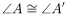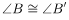and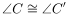.

In drawing the triangles, you made sure that the ratio of the lengths of corresponding sides is equal. In other words, you made the length of the corresponding sides proportional. For instance, the ratio of the lengths of the corresponding sides of to those of is 2. That is,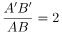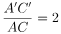and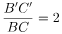Two triangles whose corresponding angles are congruent are called similar triangles. The lengths of the corresponding sides of similar triangles are proportional.

### Task 1 - Drawing Similar Triangles (Part 1)

In the applet below, given ∆ABC, use the Segment tool to draw ∆A'B'C' such that A'B' = 2AB, A'C'= 2AC, and B'C'= 2BC.

Questions

1.) How did you draw ∆A'B'C'?

2.) What do you observe about the angles of the two triangles?

3.) Draw other triangles satisfying the same conditions and see if your observations hold.

Note

In your lesson on Triangle Congruence, you have learned about corresponding angles and corresponding sides. In the two triangles above, the pairs of corresponding angles are A and A’, B and B’, and C and C’. The pairs of corresponding sides are AB and A’B’, BC and B’C’ and AC and A’C’.

## Sunday, March 19, 2017

### Visual Proof of Pythagorean Theorem

Shown below is a triangle whose sides form squares with sides a, b, and c.

1.) Move slider p to the extreme right.

What is the relationship among the squares when p was on the extreme left and the quadrilaterals when p was on the extreme right?
2.) Move slider q to the extreme right.

What is the relationship among the quadrilaterals when q was on the extreme left and when q was on the extreme right?

3.) Move slider r to the extreme right.

What is the relationship among the quadrilaterals when r was on the extreme left and when r was on the extreme right?

4.) Based on your exploration, what is the relationship among the areas of the squares with side lengths a, b, and c?

5.) Using the variables, a, b, and c write an equation about the relationship you found in 4.